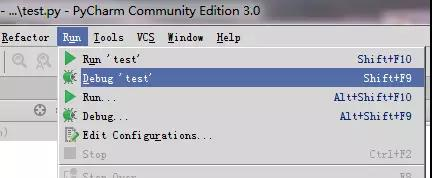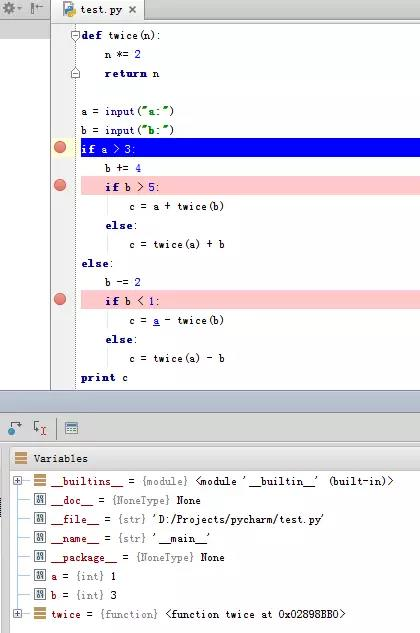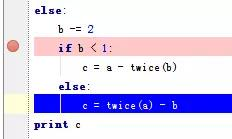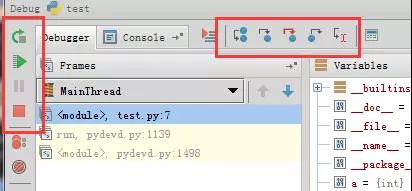# 在 Python 中使用断点调试

``````def twice(n):
n *= 2
return n
a = input("a:")
b = input("b:")
if a > 3:
b += 4
if b > 5:
c = a + twice(b)
else:
c = twice(a) + b
else:
b -= 2
if b < 1:
c = a - twice(b)
else:
c = twice(a) - b
print (c)
``````

``````def twice(n):
n *= 2
return n
a = input("a:")
b = input("b:")
print "====a,b:", a, b
if a > 3:
b += 4
print( "===1 b:", b)
if b > 5:
c = a + twice(b)
print( "====1 c:", c)

else:
c = twice(a) + b
print( "====2 c:", c)
else:
b -= 2
print( "====2 b:", b)
if b < 1:
c = a - twice(b)
print( "====3 c:", c)
else:
c = twice(a) - b
print ("====4 c:", c)
print (c)
``````

``````a:2
b:4
====a,b: 2 4
====2 b: 2
====4 c: 2
2
``````